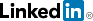cancel
Showing results for
Did you mean:
RogerbijFrequent Visitor

## Filter out row using Table.SelectRows and conditional if/and

Hi all,

I need to drop a row from my table in power query (Excel). I presume I need to filter that row out. The trouble is that other rows also have than same value and I dont want to filter out the other ones too. For example, I want to filter out

[Total]<>26 where in the same row the [Indicator] is "bob"

I do not want to filter out [Total]<>26 for "sally" and "jane"

I do not want to filter out "bob" if total does not equal 26 (since bob has other rows in other years I want to keep

I tried this

#"myfilter" = Table.SelectRows(#"previous step", each ([Total] <> 26 and [Indicator] <>"bob"))

But of course this filtered out all the 26 and all the bob entries...How do I write this please?

Best, Roger

2 ACCEPTED SOLUTIONS

Accepted SolutionsSuper User

## Re: Filter out row using Table.SelectRows and conditional if/and

You can filter to Bob and 26 and add not like below.

`= Table.SelectRows(#"Changed Type", each not ([Indicator] = "bob" and [Total] = 26))`
Best Regards,
Mariusz

If this post helps, then please consider Accepting it as the solution.

Please feel free to connect with me.Super User

## Re: Filter out row using Table.SelectRows and conditional if/and

You can try the below.

```= Table.SelectRows(#"Changed Type",
each not (
( [Indicator] = "bob" and [Total] = 26 )
or ( [Indicator] = "jane" and [Total] = 21 )
or ( [Indicator] = "jon" and [Total] = 15 )
)
)```

Best Regards,
Mariusz

If this post helps, then please consider Accepting it as the solution.

Please feel free to connect with me.5 REPLIES 5Super User

## Re: Filter out row using Table.SelectRows and conditional if/and

You can filter to Bob and 26 and add not like below.

`= Table.SelectRows(#"Changed Type", each not ([Indicator] = "bob" and [Total] = 26))`
Best Regards,
Mariusz

If this post helps, then please consider Accepting it as the solution.

Please feel free to connect with me.RogerbijFrequent Visitor

## Re: Filter out row using Table.SelectRows and conditional if/and

Thanks a lot @Mariusz ,

each not() was exactly what I needed.

I also figured I could add an Index column and then filter out that unique ID.

Cheers, Roger

RogerbijFrequent Visitor

## Re: Filter out row using Table.SelectRows and conditional if/and

Hi, can I ask a further question on this,

How can I adjust the code so that this code:

`= Table.SelectRows(#"Changed Type", each not ([Indicator] = "bob" and [Total] = 26))= Table.SelectRows(#"Changed Type", each not ([Indicator] = "jane" and [Total] = 21))= Table.SelectRows(#"Changed Type", each not ([Indicator] = "jon" and [Total] = 15))`

is wrapped in something like a

`each [indicator]each if thenelse if thenelse`

So I dont have to keep writing '= Table.SelectRows(#' ?

Thanks, RogerSuper User

## Re: Filter out row using Table.SelectRows and conditional if/and

You can try the below.

```= Table.SelectRows(#"Changed Type",
each not (
( [Indicator] = "bob" and [Total] = 26 )
or ( [Indicator] = "jane" and [Total] = 21 )
or ( [Indicator] = "jon" and [Total] = 15 )
)
)```

Best Regards,
Mariusz

If this post helps, then please consider Accepting it as the solution.

Please feel free to connect with me.Highlighted
RogerbijFrequent Visitor

## Re: Filter out row using Table.SelectRows and conditional if/and

Great, thanks! That simplifies my code a little 🙂